INSTRUCTORSCarleen EatonGrant Fraser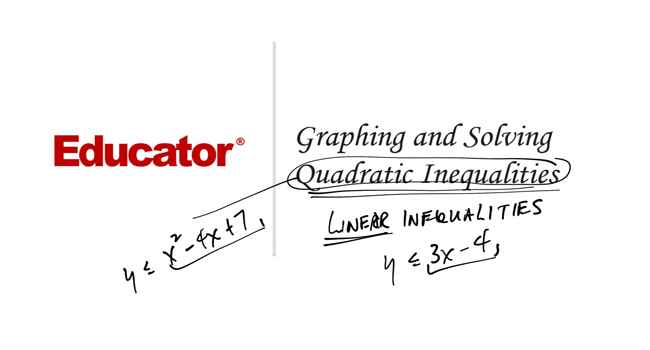Dr. Carleen Eaton

Slide Duration:

Section 1: Equations and Inequalities
Expressions and Formulas

22m 23s

Intro
0:00
Order of Operations
0:19
Variable
0:27
Algebraic Expression
0:46
Term
0:57
Example: Algebraic Expression
1:25
Evaluate Inside Grouping Symbols
1:55
Evaluate Powers
2:30
Multiply/Divide Left to Right
2:55
3:35
Monomials
4:40
Examples of Monomials
4:52
Constant
5:27
Coefficient
5:46
Degree
6:25
Power
7:15
Polynomials
8:02
Examples of Polynomials
8:24
Binomials, Trinomials, Monomials
8:53
Term
9:21
Like Terms
10:02
Formulas
11:00
Example: Pythagorean Theorem
11:15
Example 1: Evaluate the Algebraic Expression
11:50
Example 2: Evaluate the Algebraic Expression
14:38
Example 3: Area of a Triangle
19:11
Example 4: Fahrenheit to Celsius
20:41
Properties of Real Numbers

20m 15s

Intro
0:00
Real Numbers
0:07
Number Line
0:15
Rational Numbers
0:46
Irrational Numbers
2:24
Venn Diagram of Real Numbers
4:03
Irrational Numbers
5:00
Rational Numbers
5:19
Real Number System
5:27
Natural Numbers
5:32
Whole Numbers
5:53
Integers
6:19
Fractions
6:46
Properties of Real Numbers
7:15
Commutative Property
7:34
Associative Property
8:07
Identity Property
9:04
Inverse Property
9:53
Distributive Property
11:03
Example 1: What Set of Numbers?
12:21
Example 2: What Properties Are Used?
13:56
Example 3: Multiplicative Inverse
16:00
Example 4: Simplify Using Properties
17:18
Solving Equations

19m 10s

Intro
0:00
Translations
0:06
Verbal Expressions and Algebraic Expressions
0:13
Example: Sum of Two Numbers
0:19
Example: Square of a Number
1:33
Properties of Equality
3:20
Reflexive Property
3:30
Symmetric Property
3:42
Transitive Property
4:01
5:01
Subtraction Property
5:37
Multiplication Property
6:02
Division Property
6:30
Solving Equations
6:58
Example: Using Properties
7:18
Solving for a Variable
8:25
Example: Solve for Z
8:34
Example 1: Write Algebraic Expression
10:15
Example 2: Write Verbal Expression
11:31
Example 3: Solve the Equation
14:05
Example 4: Simplify Using Properties
17:26
Solving Absolute Value Equations

17m 31s

Intro
0:00
Absolute Value Expressions
0:09
Distance from Zero
0:18
Example: Absolute Value Expression
0:24
Absolute Value Equations
1:50
Example: Absolute Value Equation
2:00
Example: Isolate Expression
3:13
No Solution
3:46
Empty Set
3:58
Example: No Solution
4:12
Number of Solutions
4:46
Check Each Solution
4:57
Example: Two Solutions
5:05
Example: No Solution
6:18
Example: One Solution
6:28
Example 1: Evaluate for X
7:16
Example 2: Write Verbal Expression
9:08
Example 3: Solve the Equation
12:18
Example 4: Simplify Using Properties
13:36
Solving Inequalities

17m 14s

Intro
0:00
Properties of Inequalities
0:08
0:17
Example: Using Numbers
0:30
Subtraction Property
1:03
Example: Using Numbers
1:19
Multiplication Properties
1:44
C>0 (Positive Number)
1:50
Example: Using Numbers
2:05
C<0 (Negative Number)
2:40
Example: Using Numbers
3:10
Division Properties
4:11
C>0 (Positive Number)
4:15
Example: Using Numbers
4:27
C<0 (Negative Number)
5:21
Example: Using Numbers
5:32
Describing the Solution Set
6:10
Example: Set Builder Notation
6:26
Example: Graph (Closed Circle)
7:08
Example: Graph (Open Circle)
7:30
Example 1: Solve the Inequality
7:58
Example 2: Solve the Inequality
9:06
Example 3: Solve the Inequality
10:10
Example 4: Solve the Inequality
13:12
Solving Compound and Absolute Value Inequalities

25m

Intro
0:00
Compound Inequalities
0:08
And and Or
0:13
Example: And
0:22
Example: Or
1:12
And Inequality
1:41
Intersection
1:49
Example: Numbers
2:08
Example: Inequality
2:43
Or Inequality
4:35
Example: Union
4:45
Example: Inequality
5:53
Absolute Value Inequalities
7:19
Definition of Absolute Value
7:33
Examples: Compound Inequalities
8:30
Example: Complex Inequality
12:21
Example 1: Solve the Inequality
12:54
Example 2: Solve the Inequality
17:21
Example 3: Solve the Inequality
18:54
Example 4: Solve the Inequality
22:15
Section 2: Linear Relations and Functions
Relations and Functions

32m 5s

Intro
0:00
Coordinate Plane
0:20
X-Coordinate and Y-Coordinate
0:30
Example: Coordinate Pairs
0:37
1:20
Relations
2:14
Domain and Range
2:19
Set of Ordered Pairs
2:29
As a Table
2:51
Functions
4:21
One Element in Range
4:32
Example: Mapping
4:43
Example: Table and Map
6:26
One-to-One Functions
8:01
Example: One-to-One
8:22
Example: Not One-to-One
9:18
Graphs of Relations
11:01
Discrete and Continuous
11:12
Example: Discrete
11:22
Example: Continous
12:30
Vertical Line Test
14:09
Example: S Curve
14:29
Example: Function
16:15
Equations, Relations, and Functions
17:03
Independent Variable and Dependent Variable
17:16
Function Notation
19:11
Example: Function Notation
19:23
Example 1: Domain and Range
20:51
Example 2: Discrete or Continous
23:03
Example 3: Discrete or Continous
25:53
Example 4: Function Notation
30:05
Linear Equations

14m 46s

Intro
0:00
Linear Equations and Functions
0:07
Linear Equation
0:19
Example: Linear Equation
0:29
Example: Linear Function
1:07
Standard Form
2:02
Integer Constants with No Common Factor
2:08
Example: Standard Form
2:27
Graphing with Intercepts
4:05
X-Intercept and Y-Intercept
4:12
Example: Intercepts
4:26
Example: Graphing
5:14
Example 1: Linear Function
7:53
Example 2: Linear Function
9:10
Example 3: Standard Form
10:04
Example 4: Graph with Intercepts
12:25
Slope

23m 7s

Intro
0:00
Definition of Slope
0:07
Change in Y / Change in X
0:26
Example: Slope of Graph
0:37
Interpretation of Slope
3:07
Horizontal Line (0 Slope)
3:13
Vertical Line (Undefined Slope)
4:52
Rises to Right (Positive Slope)
6:36
Falls to Right (Negative Slope)
6:53
Parallel Lines
7:18
Example: Not Vertical
7:30
Example: Vertical
7:58
Perpendicular Lines
8:31
Example: Perpendicular
8:42
Example 1: Slope of Line
10:32
Example 2: Graph Line
11:45
Example 3: Parallel to Graph
13:37
Example 4: Perpendicular to Graph
17:57
Writing Linear Functions

23m 5s

Intro
0:00
Slope Intercept Form
0:11
m and b
0:28
Example: Graph Using Slope Intercept
0:43
Point Slope Form
2:41
Relation to Slope Formula
3:03
Example: Point Slope Form
4:36
Parallel and Perpendicular Lines
6:28
Review of Parallel and Perpendicular Lines
6:31
Example: Parallel
7:50
Example: Perpendicular
9:58
Example 1: Slope Intercept Form
11:07
Example 2: Slope Intercept Form
13:07
Example 3: Parallel
15:49
Example 4: Perpendicular
18:42
Special Functions

31m 5s

Intro
0:00
Step Functions
0:07
Example: Apple Prices
0:30
Absolute Value Function
4:55
Example: Absolute Value
5:05
Piecewise Functions
9:08
Example: Piecewise
9:27
Example 1: Absolute Value Function
14:00
Example 2: Absolute Value Function
20:39
Example 3: Piecewise Function
22:26
Example 4: Step Function
25:25
Graphing Inequalities

21m 42s

Intro
0:00
Graphing Linear Inequalities
0:07
0:19
Using Test Points
0:32
Graph Corresponding Linear Function
0:46
Dashed or Solid Lines
0:59
Use Test Point
1:21
Example: Linear Inequality
1:58
Graphing Absolute Value Inequalities
4:50
Graph Corresponding Equations
4:59
Use Test Point
5:20
Example: Absolute Value Inequality
5:38
Example 1: Linear Inequality
9:17
Example 2: Linear Inequality
11:56
Example 3: Linear Inequality
14:29
Example 4: Absolute Value Inequality
17:06
Section 3: Systems of Equations and Inequalities
Solving Systems of Equations by Graphing

17m 13s

Intro
0:00
Systems of Equations
0:09
Example: Two Equations
0:24
Solving by Graphing
0:53
Point of Intersection
1:09
Types of Systems
2:29
Independent (Single Solution)
2:34
Dependent (Infinite Solutions)
3:05
Inconsistent (No Solution)
4:23
Example 1: Solve by Graphing
5:20
Example 2: Solve by Graphing
9:10
Example 3: Solve by Graphing
12:27
Example 4: Solve by Graphing
14:54
Solving Systems of Equations Algebraically

23m 53s

Intro
0:00
Solving by Substitution
0:08
Example: System of Equations
0:36
Solving by Multiplication
7:22
Extra Step of Multiplying
7:38
Example: System of Equations
8:00
Inconsistent and Dependent Systems
11:14
Variables Drop Out
11:48
Inconsistent System (Never True)
12:01
Constant Equals Constant
12:53
Dependent System (Always True)
13:11
Example 1: Solve Algebraically
13:58
Example 2: Solve Algebraically
15:52
Example 3: Solve Algebraically
17:54
Example 4: Solve Algebraically
21:40
Solving Systems of Inequalities By Graphing

27m 12s

Intro
0:00
Solving by Graphing
0:08
Graph Each Inequality
0:25
Overlap
0:35
Corresponding Linear Equations
1:03
Test Point
1:23
Example: System of Inequalities
1:51
No Solution
7:06
Empty Set
7:26
Example: No Solution
7:34
Example 1: Solve by Graphing
10:27
Example 2: Solve by Graphing
13:30
Example 3: Solve by Graphing
17:19
Example 4: Solve by Graphing
23:23
Solving Systems of Equations in Three Variables

28m 53s

Intro
0:00
Solving Systems in Three Variables
0:17
Triple of Values
0:31
Example: Three Variables
0:56
Number of Solutions
5:55
One Solution
6:08
No Solution
6:24
Infinite Solutions
7:06
Example 1: Solve 3 Variables
7:59
Example 2: Solve 3 Variables
13:50
Example 3: Solve 3 Variables
19:54
Example 4: Solve 3 Variables
25:50
Section 4: Matrices
Basic Matrix Concepts

11m 34s

Intro
0:00
What is a Matrix
0:26
Brackets
0:46
Designation
1:21
Element
1:47
Matrix Equations
1:59
Dimensions
2:27
Rows (m) and Columns (n)
2:37
Examples: Dimensions
2:43
Special Matrices
4:22
Row Matrix
4:32
Column Matrix
5:00
Zero Matrix
6:00
Equal Matrices
6:30
Example: Corresponding Elements
6:36
Example 1: Matrix Dimension
8:12
Example 2: Matrix Dimension
9:03
Example 3: Zero Matrix
9:38
Example 4: Row and Column Matrix
10:26
Matrix Operations

21m 36s

Intro
0:00
0:18
Same Dimensions
0:25
1:04
Matrix Subtraction
3:42
Same Dimensions
3:48
Example: Subtracting Matrices
4:04
Scalar Multiplication
6:08
Scalar Constant
6:24
Example: Multiplying Matrices
6:32
Properties of Matrix Operations
8:23
Commutative Property
8:41
Associative Property
9:08
Distributive Property
9:44
10:24
Example 2: Matrix Subtraction
11:58
Example 3: Scalar Multiplication
14:23
Example 4: Matrix Properties
16:09
Matrix Multiplication

29m 36s

Intro
0:00
Dimension Requirement
0:17
n = p
0:24
Resulting Product Matrix (m x q)
1:21
Example: Multiplication
1:54
Matrix Multiplication
3:38
Example: Matrix Multiplication
4:07
Properties of Matrix Multiplication
10:46
Associative Property
11:00
Associative Property (Scalar)
11:28
Distributive Property
12:06
Distributive Property (Scalar)
12:30
Example 1: Possible Matrices
13:31
Example 2: Multiplying Matrices
17:08
Example 3: Multiplying Matrices
20:41
Example 4: Matrix Properties
24:41
Determinants

33m 13s

Intro
0:00
What is a Determinant
0:13
Square Matrices
0:23
Vertical Bars
0:41
Determinant of a 2x2 Matrix
1:21
Second Order Determinant
1:37
Formula
1:45
Example: 2x2 Determinant
1:58
Determinant of a 3x3 Matrix
2:50
Expansion by Minors
3:08
Third Order Determinant
3:19
Expanding Row One
4:06
Example: 3x3 Determinant
6:40
Diagonal Method for 3x3 Matrices
13:24
Example: Diagonal Method
13:36
Example 1: Determinant of 2x2
18:59
Example 2: Determinant of 3x3
20:03
Example 3: Determinant of 3x3
25:35
Example 4: Determinant of 3x3
29:22
Cramer's Rule

28m 25s

Intro
0:00
System of Two Equations in Two Variables
0:16
One Variable
0:50
Determinant of Denominator
1:14
Determinants of Numerators
2:23
Example: System of Equations
3:34
System of Three Equations in Three Variables
7:06
Determinant of Denominator
7:17
Determinants of Numerators
7:52
Example 1: Two Equations
8:57
Example 2: Two Equations
13:21
Example 3: Three Equations
17:11
Example 4: Three Equations
23:43
Identity and Inverse Matrices

22m 25s

Intro
0:00
Identity Matrix
0:13
Example: 2x2 Identity Matrix
0:30
Example: 4x4 Identity Matrix
0:50
Properties of Identity Matrices
1:24
Example: Multiplying Identity Matrix
2:52
Matrix Inverses
5:30
Writing Matrix Inverse
6:07
Inverse of a 2x2 Matrix
6:39
Example: 2x2 Matrix
7:31
Example 1: Inverse Matrix
10:18
Example 2: Find the Inverse Matrix
13:04
Example 3: Find the Inverse Matrix
17:53
Example 4: Find the Inverse Matrix
20:44
Solving Systems of Equations Using Matrices

22m 32s

Intro
0:00
Matrix Equations
0:11
Example: System of Equations
0:21
Solving Systems of Equations
4:01
Isolate x
4:16
Example: Using Numbers
5:10
Multiplicative Inverse
5:54
Example 1: Write as Matrix Equation
7:18
Example 2: Use Matrix Equations
9:12
Example 3: Use Matrix Equations
15:06
Example 4: Use Matrix Equations
19:35
Section 5: Quadratic Functions and Inequalities

31m 48s

Intro
0:00
0:12
A is Zero
0:27
Example: Parabola
0:45
Properties of Parabolas
2:08
Axis of Symmetry
2:11
Vertex
2:32
Example: Parabola
2:48
Minimum and Maximum Values
9:02
Positive or Negative
9:28
Upward or Downward
9:58
Example: Minimum
10:31
Example: Maximum
11:16
Example 1: Axis of Symmetry, Vertex, Graph
12:41
Example 2: Axis of Symmetry, Vertex, Graph
17:25
Example 3: Minimum or Maximum
21:47
Example 4: Minimum or Maximum
27:09

27m 3s

Intro
0:00
0:16
Standard Form
0:18
0:47
Solving by Graphing
1:41
Roots (x-Intercepts)
1:48
Example: Number of Solutions
2:12
Estimating Solutions
9:23
Example: Integer Solutions
9:30
Example: Estimating
9:53
Example 1: Solve by Graphing
10:52
Example 2: Solve by Graphing
15:10
Example 1: Solve by Graphing
17:50
Example 1: Solve by Graphing
20:54

19m 53s

Intro
0:00
Factoring Techniques
0:15
Greatest Common Factor (GCF)
0:37
Difference of Two Squares
1:48
Perfect Square Trinomials
2:30
General Trinomials
3:09
Zero Product Rule
5:22
Example: Zero Product
5:53
Example 1: Solve by Factoring
7:46
Example 1: Solve by Factoring
9:48
Example 1: Solve by Factoring
12:34
Example 1: Solve by Factoring
15:28
Imaginary and Complex Numbers

35m 45s

Intro
0:00
Properties of Square Roots
0:10
Product Property
0:26
Example: Product Property
0:56
Quotient Property
2:17
Example: Quotient Property
2:35
Imaginary Numbers
3:12
Imaginary i
3:51
Examples: Imaginary Number
4:22
Complex Numbers
7:23
Real Part and Imaginary Part
7:33
Examples: Complex Numbers
7:57
Equality
9:37
Example: Equal Complex Numbers
9:52
10:12
10:25
Complex Plane
13:32
Horizontal Axis (Real)
13:49
Vertical Axis (Imaginary)
13:59
Example: Labeling
14:11
Multiplication
15:57
Example: FOIL Method
16:03
Division
18:37
Complex Conjugates
18:45
Conjugate Pairs
19:10
Example: Dividing Complex Numbers
20:00
Example 1: Simplify Complex Number
24:50
Example 2: Simplify Complex Number
27:56
Example 3: Multiply Complex Numbers
29:27
Example 3: Dividing Complex Numbers
31:48
Completing the Square

27m 11s

Intro
0:00
Square Root Property
0:12
Example: Perfect Square
0:38
Example: Perfect Square Trinomial
3:00
Completing the Square
4:39
Constant Term
4:50
Example: Complete the Square
5:04
Solve Equations
6:42
6:59
Example: Complete the Square
7:07
Equations Where a Not Equal to 1
10:58
Divide by Coefficient
11:08
Example: Complete the Square
11:24
Complex Solutions
14:05
Real and Imaginary
14:14
Example: Complex Solution
14:35
Example 1: Square Root Property
18:31
Example 2: Complete the Square
19:15
Example 3: Complete the Square
20:40
Example 4: Complete the Square
23:56

22m 48s

Intro
0:00
0:21
Standard Form
0:29
0:57
One Rational Root
3:00
Example: One Root
3:31
Complex Solutions
6:16
Complex Conjugate
6:28
Example: Complex Solution
7:15
Discriminant
9:42
Positive Discriminant
10:03
Perfect Square (Rational)
10:51
Not Perfect Square (2 Irrational)
11:27
Negative Discriminant
12:28
Zero Discriminant
12:57
13:50
16:03
19:00
Example 4: Discriminant
21:33
Analyzing the Graphs of Quadratic Functions

30m 7s

Intro
0:00
Vertex Form
0:12
H and K
0:32
Axis of Symmetry
0:36
Vertex
0:42
Example: Origin
1:00
Example: k = 2
2:12
Example: h = 1
4:27
Significance of Coefficient a
7:13
Example: |a| > 1
7:25
Example: |a| < 1
8:18
Example: |a| > 0
8:51
Example: |a| < 0
9:05
Writing Quadratic Equations in Vertex Form
10:22
Standard Form to Vertex Form
10:35
Example: Standard Form
11:02
Example: a Term Not 1
14:42
Example 1: Vertex Form
19:47
Example 2: Vertex Form
22:09
Example 3: Vertex Form
24:32
Example 4: Vertex Form
28:23

27m 5s

Intro
0:00
0:11
Test Point
0:18
0:29
3:57
Example: Parameter
4:24
Example 1: Graph Inequality
11:16
Example 2: Solve Inequality
14:27
Example 3: Graph Inequality
19:14
Example 4: Solve Inequality
23:48
Section 6: Polynomial Functions
Properties of Exponents

19m 29s

Intro
0:00
Simplifying Exponential Expressions
0:09
Monomial Simplest Form
0:19
Negative Exponents
1:07
Examples: Simple
1:34
Properties of Exponents
3:06
Negative Exponents
3:13
Mutliplying Same Base
3:24
Dividing Same Base
3:45
Raising Power to a Power
4:33
Parentheses (Multiplying)
5:11
Parentheses (Dividing)
5:47
Raising to 0th Power
6:15
Example 1: Simplify Exponents
7:59
Example 2: Simplify Exponents
10:41
Example 3: Simplify Exponents
14:11
Example 4: Simplify Exponents
18:04
Operations on Polynomials

13m 27s

Intro
0:00
0:13
Like Terms and Like Monomials
0:23
1:14
Multiplying Polynomials
3:40
Distributive Property
3:44
Example: Monomial by Polynomial
4:06
Example 1: Simplify Polynomials
5:47
Example 2: Simplify Polynomials
6:28
Example 3: Simplify Polynomials
8:38
Example 4: Simplify Polynomials
10:47
Dividing Polynomials

31m 11s

Intro
0:00
Dividing by a Monomial
0:13
Example: Numbers
0:26
Example: Polynomial by a Monomial
1:18
Long Division
2:28
Remainder Term
2:41
Example: Dividing with Numbers
3:04
Example: With Polynomials
5:01
Example: Missing Terms
7:58
Synthetic Division
11:44
Restriction
12:04
Example: Divisor in Form
12:20
Divisor in Synthetic Division
15:54
Example: Coefficient to 1
16:07
Example 1: Divide Polynomials
17:10
Example 2: Divide Polynomials
19:08
Example 3: Synthetic Division
21:42
Example 4: Synthetic Division
25:09
Polynomial Functions

22m 30s

Intro
0:00
Polynomial in One Variable
0:13
0:27
Example: Polynomial
1:18
Degree
1:31
Polynomial Functions
2:57
Example: Function
3:13
Function Values
3:33
Example: Numerical Values
3:53
Example: Algebraic Expressions
5:11
Zeros of Polynomial Functions
5:50
Odd Degree
6:04
Even Degree
7:29
End Behavior
8:28
Even Degrees
9:09
9:23
Odd Degrees
12:51
13:00
Example 1: Degree and Leading Coefficient
15:03
Example 2: Polynomial Function
15:56
Example 3: Polynomial Function
17:34
Example 4: End Behavior
19:53
Analyzing Graphs of Polynomial Functions

33m 29s

Intro
0:00
Graphing Polynomial Functions
0:11
Example: Table and End Behavior
0:39
Location Principle
4:43
Zero Between Two Points
5:03
Example: Location Principle
5:21
Maximum and Minimum Points
8:40
Relative Maximum and Relative Minimum
9:16
Example: Number of Relative Max/Min
11:11
Example 1: Graph Polynomial Function
11:57
Example 2: Graph Polynomial Function
16:19
Example 3: Graph Polynomial Function
23:27
Example 4: Graph Polynomial Function
28:35
Solving Polynomial Functions

21m 10s

Intro
0:00
Factoring Polynomials
0:06
Greatest Common Factor (GCF)
0:25
Difference of Two Squares
1:14
Perfect Square Trinomials
2:07
General Trinomials
2:57
Grouping
4:32
Sum and Difference of Two Cubes
6:03
Examples: Two Cubes
6:14
8:22
8:44
Example 1: Factor Polynomial
12:03
Example 2: Factor Polynomial
13:54
15:33
Example 4: Solve Polynomial Function
17:24
Remainder and Factor Theorems

31m 21s

Intro
0:00
Remainder Theorem
0:07
Checking Work
0:22
Dividend and Divisor in Theorem
1:12
Example: f(a)
2:05
Synthetic Substitution
5:43
Example: Polynomial Function
6:15
Factor Theorem
9:54
Example: Numbers
10:16
Example: Confirm Factor
11:27
Factoring Polynomials
14:48
Example: 3rd Degree Polynomial
15:07
Example 1: Remainder Theorem
19:17
Example 2: Other Factors
21:57
Example 3: Remainder Theorem
25:52
Example 4: Other Factors
28:21
Roots and Zeros

31m 27s

Intro
0:00
Number of Roots
0:08
Not Nature of Roots
0:18
Example: Real and Complex Roots
0:25
Descartes' Rule of Signs
2:05
Positive Real Roots
2:21
Example: Positve
2:39
Negative Real Roots
5:44
Example: Negative
6:06
Finding the Roots
9:59
Example: Combination of Real and Complex
10:07
Conjugate Roots
13:18
Example: Conjugate Roots
13:50
Example 1: Solve Polynomial
16:03
Example 2: Solve Polynomial
18:36
Example 3: Possible Combinations
23:13
Example 4: Possible Combinations
27:11
Rational Zero Theorem

31m 16s

Intro
0:00
Equation
0:08
List of Possibilities
0:16
Equation with Constant and Leading Coefficient
1:04
Example: Rational Zero
2:46
7:19
Equation with Leading Coefficient of One
7:34
Example: Coefficient Equal to 1
8:45
Finding Rational Zeros
12:58
Division with Remainder Zero
13:32
Example 1: Possible Rational Zeros
14:20
Example 2: Possible Rational Zeros
16:02
Example 3: Possible Rational Zeros
19:58
Example 4: Find All Zeros
22:06
Section 7: Radical Expressions and Inequalities
Operations on Functions

34m 30s

Intro
0:00
Arithmetic Operations
0:07
Domain
0:16
Intersection
0:24
Denominator is Zero
0:49
Example: Operations
1:02
Composition of Functions
7:18
Notation
7:48
Right to Left
8:18
Example: Composition
8:48
Composition is Not Commutative
17:23
Example: Not Commutative
17:51
Example 1: Function Operations
20:55
Example 2: Function Operations
24:34
Example 3: Compositions
27:51
Example 4: Function Operations
31:09
Inverse Functions and Relations

22m 42s

Intro
0:00
Inverse of a Relation
0:14
Example: Ordered Pairs
0:56
Inverse of a Function
3:24
Domain and Range Switched
3:52
Example: Inverse
4:28
Procedure to Construct an Inverse Function
6:42
f(x) to y
6:42
Interchange x and y
6:59
Solve for y
7:06
Write Inverse f(x) for y
7:14
Example: Inverse Function
7:25
Example: Inverse Function 2
8:48
Inverses and Compositions
10:44
Example: Inverse Composition
11:46
Example 1: Inverse Relation
14:49
Example 2: Inverse of Function
15:40
Example 3: Inverse of Function
17:06
Example 4: Inverse Functions
18:55
Square Root Functions and Inequalities

30m 4s

Intro
0:00
Square Root Functions
0:07
Examples: Square Root Function
0:16
Example: Not Square Root Function
0:46
1:12
Example: Restriction
1:31
Graphing Square Root Functions
3:42
Example: Graphing
3:49
Square Root Inequalities
8:47
Same Technique
9:00
Example: Square Root Inequality
9:20
Example 1: Graph Square Root Function
15:19
Example 2: Graph Square Root Function
18:03
Example 3: Graph Square Root Function
22:41
Example 4: Square Root Inequalities
25:37
nth Roots

20m 46s

Intro
0:00
Definition of the nth Root
0:07
Example: 5th Root
0:20
Example: 6th Root
0:51
Principal nth Root
1:39
Example: Principal Roots
2:06
Using Absolute Values
5:58
Example: Square Root
6:18
Example: 6th Root
8:40
Example: Negative
10:15
12:23
13:29
16:07
18:18

41m 11s

Intro
0:00
0:16
Quotient Property
0:29
Example: Quotient
1:00
Example: Product Property
1:47
3:24
3:47
6:33
7:16
Rationalizing Denominators
8:27
9:05
11:47
Conjugates
12:07
13:11
16:12
16:20
16:28
19:04
Distributive Property
19:10
19:20
24:11
28:43
32:00
36:34
Rational Exponents

30m 45s

Intro
0:00
Definition 1
0:20
Example: Using Numbers
0:39
Example: Non-Negative
2:46
Example: Odd
3:34
Definition 2
4:32
Restriction
4:52
Example: Relate to Definition 1
5:04
Example: m Not 1
5:31
Simplifying Expressions
7:53
Multiplication
8:31
Division
9:29
Multiply Exponents
10:08
Raised Power
11:05
Zero Power
11:29
Negative Power
11:49
Simplified Form
13:52
Complex Fraction
14:16
Negative Exponents
14:40
Example: More Complicated
15:14
19:03
Example 2: Write with Rational Exponents
20:40
Example 3: Complex Fraction
22:09
Example 4: Complex Fraction
26:22

31m 27s

Intro
0:00
0:11
0:22
1:06
Example: Complex Equation
2:42
Extraneous Roots
7:21
Squaring Technique
7:35
Double Check
7:44
Example: Extraneous
8:21
Eliminating nth Roots
10:04
Isolate and Raise Power
10:14
Example: nth Root
10:27
11:27
Restriction: Index is Even
11:53
12:29
15:41
17:44
20:24
24:34
Section 8: Rational Equations and Inequalities
Multiplying and Dividing Rational Expressions

40m 54s

Intro
0:00
Simplifying Rational Expressions
0:22
Algebraic Fraction
0:29
Examples: Rational Expressions
0:49
Example: GCF
1:33
Example: Simplify Rational Expression
2:26
Factoring -1
4:04
Example: Simplify with -1
4:19
Multiplying and Dividing Rational Expressions
6:59
Multiplying and Dividing
7:28
Example: Multiplying Rational Expressions
8:36
Example: Dividing Rational Expressions
11:20
Factoring
14:01
Factoring Polynomials
14:19
Example: Factoring
14:35
Complex Fractions
18:22
Example: Numbers
18:37
Example: Algebraic Complex Fractions
19:25
Example 1: Simplify Rational Expression
25:56
Example 2: Simplify Rational Expression
29:34
Example 3: Simplify Rational Expression
31:39
Example 4: Simplify Rational Expression
37:50

55m 4s

Intro
0:00
Least Common Multiple (LCM)
0:27
Examples: LCM of Numbers
0:43
Example: LCM of Polynomials
4:02
7:55
Least Common Denominator (LCD)
8:07
Example: Numbers
8:17
Example: Rational Expressions
11:03
Equivalent Fractions
15:22
Simplifying Complex Fractions
21:19
Example: Previous Lessons
21:36
Example: More Complex
22:53
Example 1: Find LCM
28:30
31:44
Example 3: Subtract Rational Expressions
39:18
Example 4: Simplify Rational Expression
38:26
Graphing Rational Functions

57m 13s

Intro
0:00
Rational Functions
0:18
Restriction
0:34
Example: Rational Function
0:51
Breaks in Continuity
2:52
Example: Continuous Function
3:10
Discontinuities
3:30
Example: Excluded Values
4:37
Graphs and Discontinuities
5:02
Common Binomial Factor (Hole)
5:08
Example: Common Factor
5:31
Asymptote
10:06
Example: Vertical Asymptote
11:08
Horizontal Asymptotes
20:00
Example: Horizontal Asymptote
20:25
Example 1: Holes and Vertical Asymptotes
26:12
Example 2: Graph Rational Faction
28:35
Example 3: Graph Rational Faction
39:23
Example 4: Graph Rational Faction
47:28
Direct, Joint, and Inverse Variation

20m 21s

Intro
0:00
Direct Variation
0:07
Constant of Variation
0:25
Graph of Constant Variation
1:26
Slope is Constant k
1:35
Example: Straight Lines
1:41
Joint Variation
2:48
Three Variables
2:52
Inverse Variation
3:38
Rewritten Form
3:52
Examples in Biology
4:22
Graph of Inverse Variation
4:51
Asymptotes are Axes
5:12
Example: Inverse Variation
5:40
Proportions
10:11
Direct Variation
10:25
Inverse Variation
11:32
Example 1: Type of Variation
12:42
Example 2: Direct Variation
14:13
Example 3: Joint Variation
16:24
Example 4: Graph Rational Faction
18:50
Solving Rational Equations and Inequalities

55m 14s

Intro
0:00
Rational Equations
0:15
Example: Algebraic Fraction
0:26
Least Common Denominator
0:49
Example: Simple Rational Equation
1:22
Example: Solve Rational Equation
5:40
Extraneous Solutions
9:31
Doublecheck
10:00
No Solution
10:38
Example: Extraneous
10:44
Rational Inequalities
14:01
Excluded Values
14:31
Solve Related Equation
14:49
Find Intervals
14:58
Use Test Values
15:25
Example: Rational Inequality
15:51
Example: Rational Inequality 2
17:07
Example 1: Rational Equation
28:50
Example 2: Rational Equation
33:51
Example 3: Rational Equation
38:19
Example 4: Rational Inequality
46:49
Section 9: Exponential and Logarithmic Relations
Exponential Functions

35m 58s

Intro
0:00
What is an Exponential Function?
0:12
Restriction on b
0:31
Base
0:46
Example: Exponents as Bases
0:56
Variables as Exponents
1:12
Example: Exponential Function
1:50
Graphing Exponential Functions
2:33
Example: Using Table
2:49
Properties
11:52
Continuous and One to One
12:00
Domain is All Real Numbers
13:14
X-Axis Asymptote
13:55
Y-Intercept
14:02
Reflection Across Y-Axis
14:31
Growth and Decay
15:06
Exponential Growth
15:10
Real Life Examples
15:41
Example: Growth
15:52
Example: Decay
16:12
Real Life Examples
16:30
Equations
17:32
Bases are Same
18:05
Examples: Variables as Exponents
18:20
Inequalities
21:29
Property
21:51
Example: Inequality
22:37
Example 1: Graph Exponential Function
24:05
Example 2: Growth or Decay
27:50
Example 3: Exponential Equation
29:31
Example 4: Exponential Inequality
32:54
Logarithms and Logarithmic Functions

45m 54s

Intro
0:00
What are Logarithms?
0:08
Restrictions
0:15
Written Form
0:26
Logarithms are Exponents
0:52
Example: Logarithms
1:49
Logarithmic Functions
5:14
Same Restrictions
5:30
Inverses
5:53
Example: Logarithmic Function
6:24
Graph of the Logarithmic Function
9:20
Example: Using Table
9:35
Properties
15:09
Continuous and One to One
15:14
Domain
15:36
Range
15:56
Y-Axis is Asymptote
16:02
X Intercept
16:12
Inverse Property
16:57
Compositions of Functions
17:10
Equations
18:30
Example: Logarithmic Equation
19:13
Inequalities
20:36
Properties
20:47
Example: Logarithmic Inequality
21:40
Equations with Logarithms on Both Sides
24:43
Property
24:51
Example: Both Sides
25:23
Inequalities with Logarithms on Both Sides
26:52
Property
27:02
Example: Both Sides
28:05
Example 1: Solve Log Equation
31:52
Example 2: Solve Log Equation
33:53
Example 3: Solve Log Equation
36:15
39:19
Properties of Logarithms

28m 43s

Intro
0:00
Product Property
0:08
Example: Product
0:46
Quotient Property
2:40
Example: Quotient
2:59
Power Property
3:51
Moved Exponent
4:07
Example: Power
4:37
Equations
5:15
Example: Use Properties
5:58
Example 1: Simplify Log
11:17
Example 2: Single Log
15:54
Example 3: Solve Log Equation
18:48
Example 4: Solve Log Equation
22:13
Common Logarithms

25m 23s

Intro
0:00
What are Common Logarithms?
0:10
Real World Applications
0:16
Base Not Written
0:27
Example: Base 10
0:39
Equations
1:47
Example: Same Base
1:56
Example: Different Base
2:37
Inequalities
6:07
Multiplying/Dividing Inequality
6:21
6:54
Change of Base
12:45
Base 10
13:24
Example: Change of Base
14:05
Example 1: Log Equation
15:21
Example 2: Common Logs
17:13
Example 3: Log Equation
18:22
21:52
Base e and Natural Logarithms

21m 14s

Intro
0:00
Number e
0:09
Natural Base
0:21
Growth/Decay
0:33
Example: Exponential Function
0:53
Natural Logarithms
1:11
ln x
1:19
Inverse and Identity Function
1:39
Example: Inverse Composition
1:55
Equations and Inequalities
4:39
Extraneous Solutions
5:30
Examples: Natural Log Equations
5:48
Example 1: Natural Log Equation
9:08
Example 2: Natural Log Equation
10:37
16:54
18:16
Exponential Growth and Decay

24m 30s

Intro
0:00
Decay
0:17
Decreases by Fixed Percentage
0:23
Rate of Decay
0:56
Example: Finance
1:34
Scientific Model of Decay
3:37
Exponential Decay
3:45
4:13
Example: Half Life
5:33
Growth
9:06
Increases by Fixed Percentage
9:18
Example: Finance
10:09
Scientific Model of Growth
11:35
Population Growth
12:04
Example: Growth
12:20
Example 1: Computer Price
14:00
Example 2: Stock Price
15:46
Example 3: Medicine Disintegration
19:10
Example 4: Population Growth
22:33
Section 10: Conic Sections
Midpoint and Distance Formulas

32m 42s

Intro
0:00
Midpoint Formula
0:15
Example: Midpoint
0:30
Distance Formula
2:30
Example: Distance
2:52
Example 1: Midpoint and Distance
4:58
Example 2: Midpoint and Distance
8:07
Example 3: Median Length
18:51
Example 4: Perimeter and Area
23:36
Parabolas

41m 27s

Intro
0:00
What is a Parabola?
0:20
Definition of a Parabola
0:29
Focus
0:59
Directrix
1:15
Axis of Symmetry
3:08
Vertex
3:33
Minimum or Maximum
3:44
Standard Form
4:59
Horizontal Parabolas
5:08
Vertex Form
5:19
Upward or Downward
5:41
Example: Standard Form
6:06
Graphing Parabolas
8:31
Shifting
8:51
Example: Completing the Square
9:22
Symmetry and Translation
12:18
Example: Graph Parabola
12:40
Latus Rectum
17:13
Length
18:15
Example: Latus Rectum
18:35
Horizontal Parabolas
18:57
Not Functions
20:08
Example: Horizontal Parabola
21:21
Focus and Directrix
24:11
Horizontal
24:48
Example 1: Parabola Standard Form
25:12
Example 2: Graph Parabola
30:00
Example 3: Graph Parabola
33:13
Example 4: Parabola Equation
37:28
Circles

21m 3s

Intro
0:00
What are Circles?
0:08
Example: Equidistant
0:17
0:32
Equation of a Circle
0:44
Example: Standard Form
1:11
Graphing Circles
1:47
Example: Circle
1:56
Center Not at Origin
3:07
Example: Completing the Square
3:51
Example 1: Equation of Circle
6:44
11:51
15:08
Example 4: Equation of Circle
16:57
Ellipses

46m 51s

Intro
0:00
What Are Ellipses?
0:11
Foci
0:23
Properties of Ellipses
1:43
Major Axis, Minor Axis
1:47
Center
1:54
Length of Major Axis and Minor Axis
3:21
Standard Form
5:33
Example: Standard Form of Ellipse
6:09
Vertical Major Axis
9:14
Example: Vertical Major Axis
9:46
Graphing Ellipses
12:51
Complete the Square and Symmetry
13:00
Example: Graphing Ellipse
13:16
Equation with Center at (h, k)
19:57
Horizontal and Vertical
20:14
Difference
20:27
Example: Center at (h, k)
20:55
Example 1: Equation of Ellipse
24:05
Example 2: Equation of Ellipse
27:57
Example 3: Equation of Ellipse
32:32
Example 4: Graph Ellipse
38:27
Hyperbolas

38m 15s

Intro
0:00
What are Hyperbolas?
0:12
Two Branches
0:18
Foci
0:38
Properties
2:00
Transverse Axis and Conjugate Axis
2:06
Vertices
2:46
Length of Transverse Axis
3:14
Distance Between Foci
3:31
Length of Conjugate Axis
3:38
Standard Form
5:45
Vertex Location
6:36
Known Points
6:52
Vertical Transverse Axis
7:26
Vertex Location
7:50
Asymptotes
8:36
Vertex Location
8:56
Rectangle
9:28
Diagonals
10:29
Graphing Hyperbolas
12:58
Example: Hyperbola
13:16
Equation with Center at (h, k)
16:32
Example: Center at (h, k)
17:21
Example 1: Equation of Hyperbola
19:20
Example 2: Equation of Hyperbola
22:48
Example 3: Graph Hyperbola
26:05
Example 4: Equation of Hyperbola
36:29
Conic Sections

18m 43s

Intro
0:00
Conic Sections
0:16
Double Cone Sections
0:24
Standard Form
1:27
General Form
1:37
Identify Conic Sections
2:16
B = 0
2:50
X and Y
3:22
Identify Conic Sections, Cont.
4:46
Parabola
5:17
Circle
5:51
Ellipse
6:31
Hyperbola
7:10
Example 1: Identify Conic Section
8:01
Example 2: Identify Conic Section
11:03
Example 3: Identify Conic Section
11:38
Example 4: Identify Conic Section
14:50

47m 4s

Intro
0:00
0:22
0:45
Solutions
2:49
Graphs of Possible Solutions
3:10
4:10
Example: Elimination
4:21
Solutions
11:39
Example: 0, 1, 2, 3, 4 Solutions
11:50
12:48
13:09
21:42
29:13
35:02
40:29
Section 11: Sequences and Series
Arithmetic Sequences

21m 16s

Intro
0:00
Sequences
0:10
General Form of Sequence
0:16
Example: Finite/Infinite Sequences
0:33
Arithmetic Sequences
0:28
Common Difference
2:41
Example: Arithmetic Sequence
2:50
Formula for the nth Term
3:51
Example: nth Term
4:32
Equation for the nth Term
6:37
Example: Using Formula
6:56
Arithmetic Means
9:47
Example: Arithmetic Means
10:16
Example 1: nth Term
12:38
Example 2: Arithmetic Means
13:49
Example 3: Arithmetic Means
16:12
Example 4: nth Term
18:26
Arithmetic Series

21m 36s

Intro
0:00
What are Arithmetic Series?
0:11
Common Difference
0:28
Example: Arithmetic Sequence
0:43
Example: Arithmetic Series
1:09
Finite/Infinite Series
1:36
Sum of Arithmetic Series
2:27
Example: Sum
3:21
Sigma Notation
5:53
Index
6:14
Example: Sigma Notation
7:14
Example 1: First Term
9:00
Example 2: Three Terms
10:52
Example 3: Sum of Series
14:14
Example 4: Sum of Series
18:13
Geometric Sequences

23m 3s

Intro
0:00
Geometric Sequences
0:11
Common Difference
0:38
Common Ratio
1:08
Example: Geometric Sequence
2:38
nth Term of a Geometric Sequence
4:41
Example: nth Term
4:56
Geometric Means
6:51
Example: Geometric Mean
7:09
Example 1: 9th Term
12:04
Example 2: Geometric Means
15:18
Example 3: nth Term
18:32
Example 4: Three Terms
20:59
Geometric Series

22m 43s

Intro
0:00
What are Geometric Series?
0:11
List of Numbers
0:24
Example: Geometric Series
1:12
Sum of Geometric Series
2:16
Example: Sum of Geometric Series
2:41
Sigma Notation
4:21
Lower Index, Upper Index
4:38
Example: Sigma Notation
4:57
Another Sum Formula
6:08
Example: n Unknown
6:28
Specific Terms
7:41
Sum Formula
7:56
Example: Specific Term
8:11
Example 1: Sum of Geometric Series
10:02
Example 2: Sum of 8 Terms
14:15
Example 3: Sum of Geometric Series
18:23
Example 4: First Term
20:16
Infinite Geometric Series

18m 32s

Intro
0:00
What are Infinite Geometric Series
0:10
Example: Finite
0:29
Example: Infinite
0:51
Partial Sums
1:09
Formula
1:37
Sum of an Infinite Geometric Series
2:39
Convergent Series
2:58
Example: Sum of Convergent Series
3:28
Sigma Notation
7:31
Example: Sigma
8:17
Repeating Decimals
8:42
Example: Repeating Decimal
8:53
Example 1: Sum of Infinite Geometric Series
12:15
Example 2: Repeating Decimal
13:24
Example 3: Sum of Infinite Geometric Series
15:14
Example 4: Repeating Decimal
16:48
Recursion and Special Sequences

14m 34s

Intro
0:00
Fibonacci Sequence
0:05
Background of Fibonacci
0:23
Recursive Formula
0:37
Fibonacci Sequence
0:52
Example: Recursive Formula
2:18
Iteration
3:49
Example: Iteration
4:30
Example 1: Five Terms
7:08
Example 2: Three Terms
9:00
Example 3: Five Terms
10:38
Example 4: Three Iterates
12:41
Binomial Theorem

48m 30s

Intro
0:00
Pascal's Triangle
0:06
Expand Binomial
0:13
Pascal's Triangle
4:26
Properties
6:52
Example: Properties of Binomials
6:58
Factorials
9:11
Product
9:28
Example: Factorial
9:45
Binomial Theorem
11:08
Example: Binomial Theorem
13:48
Finding a Specific Term
18:36
Example: Specific Term
19:26
Example 1: Expand
24:39
Example 2: Fourth Term
30:26
Example 3: Five Terms
36:13
Example 4: Three Iterates
45:07
Bookmark & Share Embed

## Copy & Paste this embed code into your website’s HTML

Please ensure that your website editor is in text mode when you paste the code.
(In Wordpress, the mode button is on the top right corner.)
×
• - Allow users to view the embedded video in full-size.
Since this lesson is not free, only the preview will appear on your website.

• ## Related Books3 answersLast reply by: Merin JojySat Jan 23, 2021 12:49 PMPost by julius mogyorossy on June 18, 2015I didn't explain it as simply as I should have, about seeing where the solution set is going to be. You don't need a test point, it tells you right where it is going to be, it is obvious, it could not be more obvious. Look at example one, it say it said that y2, and one of the roots is (2,0) where x=2 and y=0. I am also confused by why you say the solution set is x<-3 and x>2, when the quadratic equation clearly says it is x>-3, x>2. From the way mathematicians look at it it seems the negative solution is a lie, and the positive one the truth. The way I find the solution set is to look at the quadratic inequality when it is in the form where the 0, the y, is all alone on the right, then it is obvious where it is, it is telling you that 0, y values for the solution set, are less than x'2+x-6, which is the graph line that you will draw, since, a, is positive and it will face up, and y values for the solution set are less than the graph line they must be below that graph line, on the outside, since it faces up, it it faced downwards, on the inside. This method I call the Julius supergenius way of finding the solution set, maybe this what you were saying when you talked about the logical way to find the solution set, if so I could not understand so.1 answerLast reply by: Dr Carleen EatonThu Jun 6, 2013 11:05 PMPost by Kavita Agrawal on June 6, 2013The last example was greater than, so the boundary should have been dotted, not solid.1 answerLast reply by: John StedgeMon Jun 4, 2018 4:19 PMPost by julius mogyorossy on March 25, 2013As soon as you see -x'2 + x + 2 >y, -x'2 + x + 2 < y, x'2 + x + 2 > y, or, x'2 + x + 2 < y, you should know instantly where the solutions are at, the equations tell you even without finding the roots, sometimes the roots are not the roots, have you noticed.1 answerLast reply by: Dr Carleen EatonTue Feb 19, 2013 7:44 PMPost by Kenneth Montfort on February 13, 2013How do you know when to combine the solutions and when not to combine the solutions? I keep hearing "this 'and' that" or "this 'or' that".1 answerLast reply by: Dr Carleen EatonMon Jul 16, 2012 7:53 PMPost by George Welborn on July 14, 2012At around 11:08 you said "at points when x is less than or equal to negative three or greater than or equal to negative two" but it should be just two, not negative two.

### Graphing and Solving Quadratic Inequalities

• Use the same techniques that were used to solve linear inequalities. Follow the conventions about how to draw the boundary curve (solid line or dotted line) and use a test point to determine the region that is the solution set. Sometimes, more than one test point must be used.

### Graphing and Solving Quadratic Inequalities

Graph the inequality y ≤ x2 + 8x + 19
• Find the vertex x = − [b/2a]
• x = − [b/2a] = − [8/2] = − 4
• f( − 4) = ( − 4)2 + 8( − 4) + 19 = 3
• Vertex = ( − 4,3)
• Locate three points on the parabola to the right of the vertex. Use property of symmetry to sketch the other half of the parabola
•  x y=x2+8x+19 -3 4 -2 7 -1 12
• Graph the parabola using the vertex and the three additional points, as well as the other points you found using property of symmetry. The line should be a solid line.
• Test which way to shade using the point (0,0) since it is not on the parabola.
•  Test Using (0,0) y ≤ x2+8x+19
•  Test Using (0,0) y ≤ x2+8x+19 0 ≤ (0)2 +8(0)+19 0 ≤ 19 True, Shade Towards (0,0)
• Shade from the line of the parabola outwards towards and including (0,0)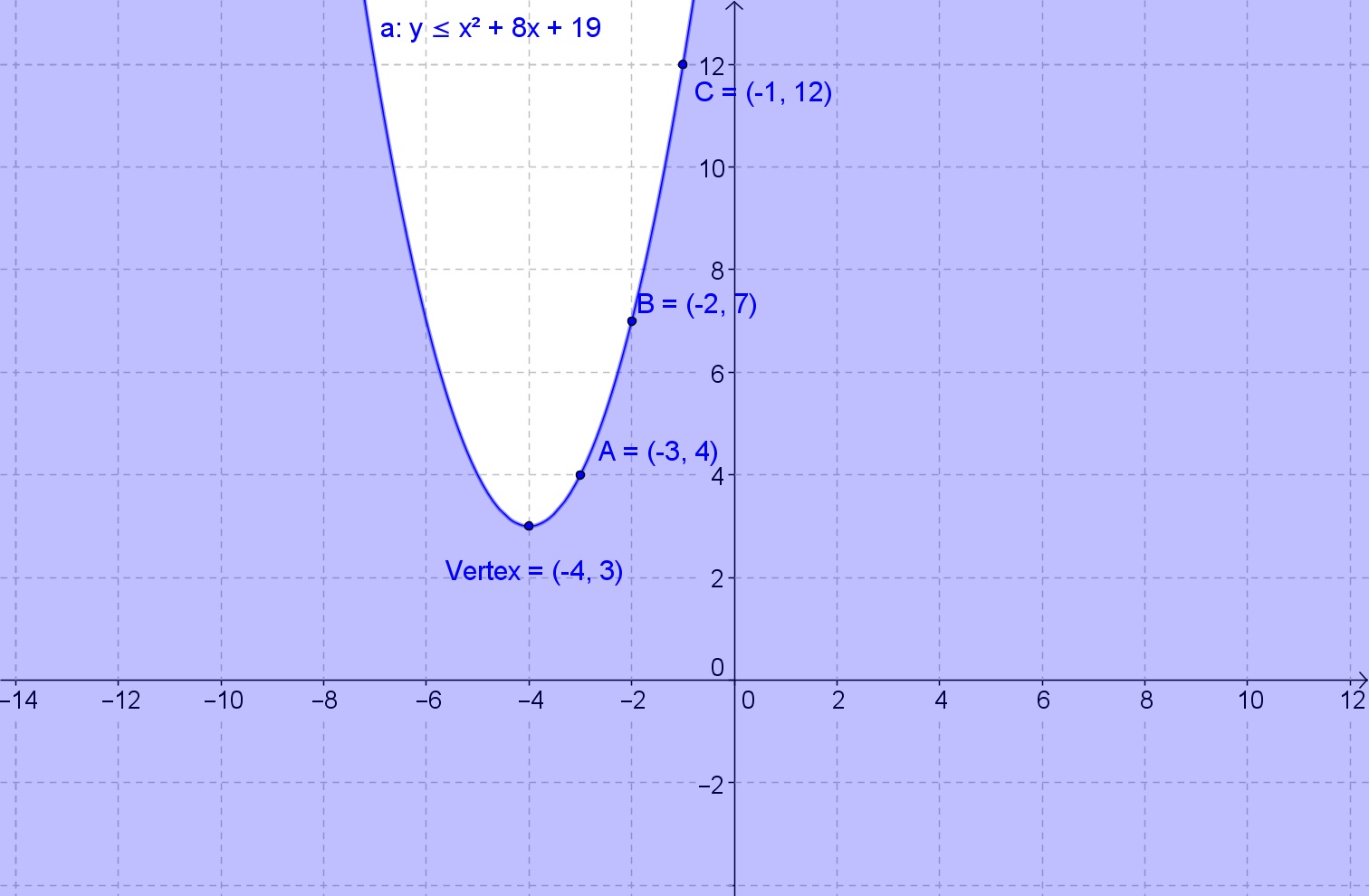Graph the inequality yx2 − 8x + 19
• Find the vertex x = − [b/2a]
• x = − [b/2a] = − [(( − 8))/2] = 4
• f(4) = (4)2 − 8(4) + 19 = 3
• Vertex = (4,3)
• Locate three points on the parabola to the right of the vertex. Use property of symmetry to sketch the other half of the parabola
•  x y=x2−8x+19 5 4 6 7 7 12
• Graph the parabola using the vertex and the three additional points, as well as the other points you found using property of symmetry. The line should be a solid line.
• Test which way to shade using the point (0,0) since it is not on the parabola.
• Test using (0,0)
• yx2 − 8x + 19
• 0 ≥ (0)2 − 8(0) + 19
• 0 ≥ 19
• Not True, shade inside the parabola only
• Shade from the line of the parabola inwards, excluding everything outside the line of the parabola including (0,0)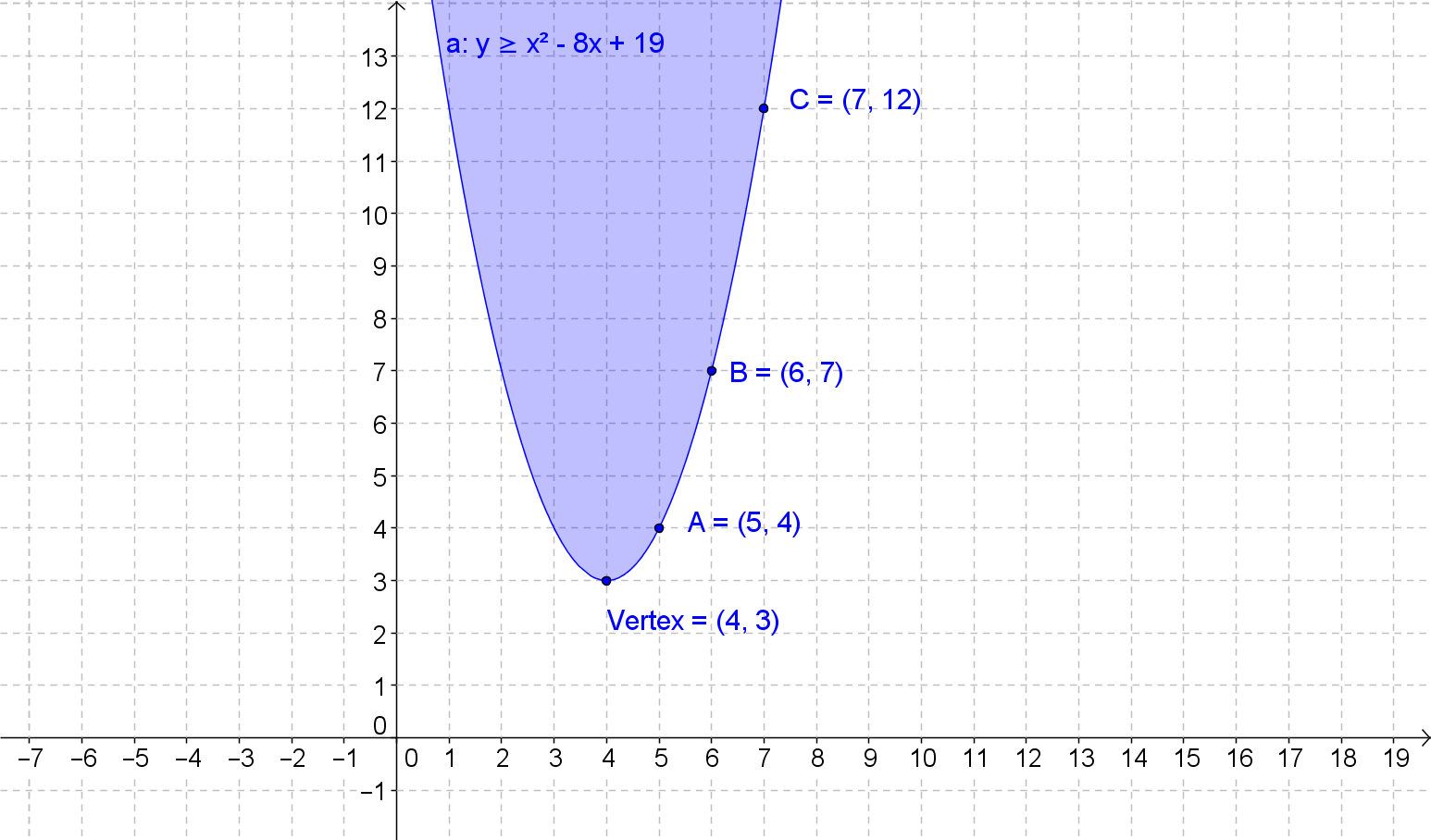Graph the inequality y < x2 − 6x + 12
• Find the vertex x = − [b/2a]
• x = − [b/2a] = − [(( − 6))/2] = 3
• f(3) = (3)2 − 6(3) + 12 = 3
• Vertex = (3,3)
• Locate three points on the parabola to the right of the vertex. Use property of symmetry to sketch the other half of the parabola
•  x y=x2−6x+12 4 4 5 7 6 12
• Graph the parabola using the vertex and the three additional points, as well as the other points you found using property of symmetry. The line should be a dashed lined because of the < sign.
• Test which way to shade using the point (0,0) since it is not on the parabola.
• Test using (0,0)
• y < x2 − 6x + 12
• 0 < (0)2 − 6(0) + 12
• 0 < 12
• True, shade outside the parabola
• Shade everything outside the parabola.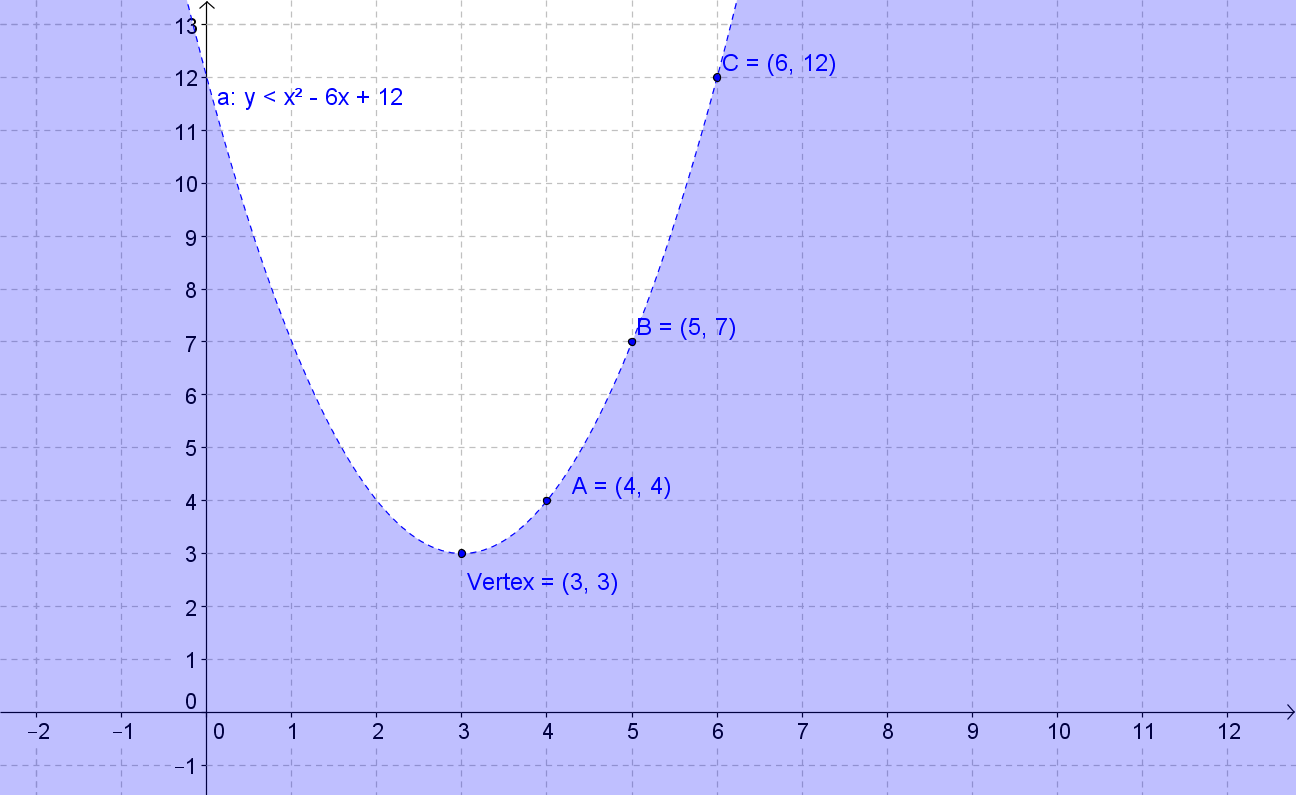Graph the inequality y < x2 + 4x + 6
• Find the vertex x = − [b/2a]
• x = − [b/2a] = − [(4)/2] = − 2
• f( − 2) = ( − 2)2 + 4( − 2) + 6 = 2
• Vertex = ( − 2,2)
• Locate three points on the parabola to the right of the vertex. Use property of symmetry to sketch the other half of the parabola
•  x y=x2−6x+12 -1 3 0 6 1 11
• Graph the parabola using the vertex and the three additional points, as well as the other points you found using property of symmetry. The line should be a dashed lined because of the < sign.
• Test which way to shade using the point (0,0) since it is not on the parabola.
• Test using (0,0)
• y < x2 + 4x + 6
• 0 < (0)2 + 4(0) + 6
• 0 < 6
• True, shade outside the parabola
• Shade everything outside the parabola.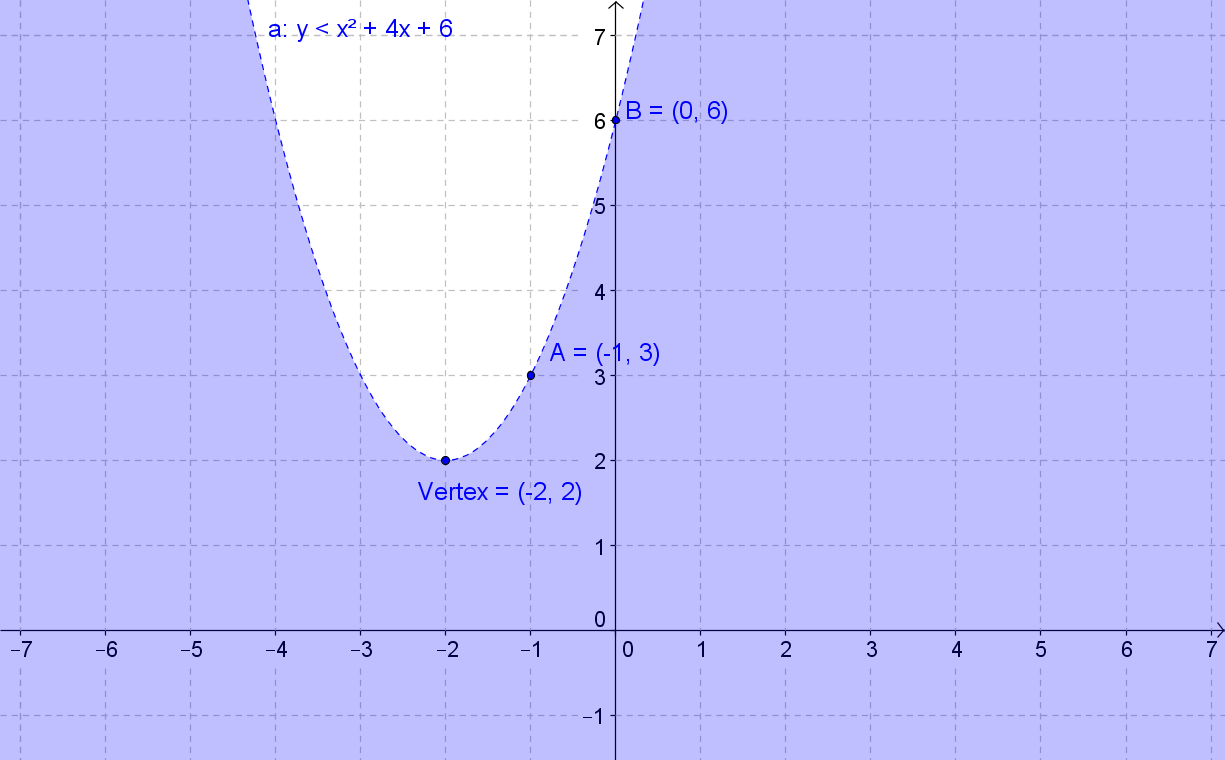Graph the inequality y > x2 + 2x + 4
• Find the vertex x = − [b/2a]
• x = − [b/2a] = − [(2)/2] = − 1
• f( − 1) = ( − 1)2 + 2( − 1) + 4 = 3
• Vertex = ( − 1,3)
• Locate three points on the parabola to the right of the vertex. Use property of symmetry to sketch the other half of the parabola
•  x y=x2+2x+4 0 4 1 7 2 12
• Graph the parabola using the vertex and the three additional points, as well as the other points you found using property of symmetry. The line should be a dashed lined because of the > sign.
• Test which way to shade using the point (0,0) since it is not on the parabola.
• Test using (0,0)
• y > x2 + 2x + 4
• 0 > (0)2 + 2(0) + 4
• 0 > 4
• Not True, shade inside the parabola
• Shade everything inside the parabola.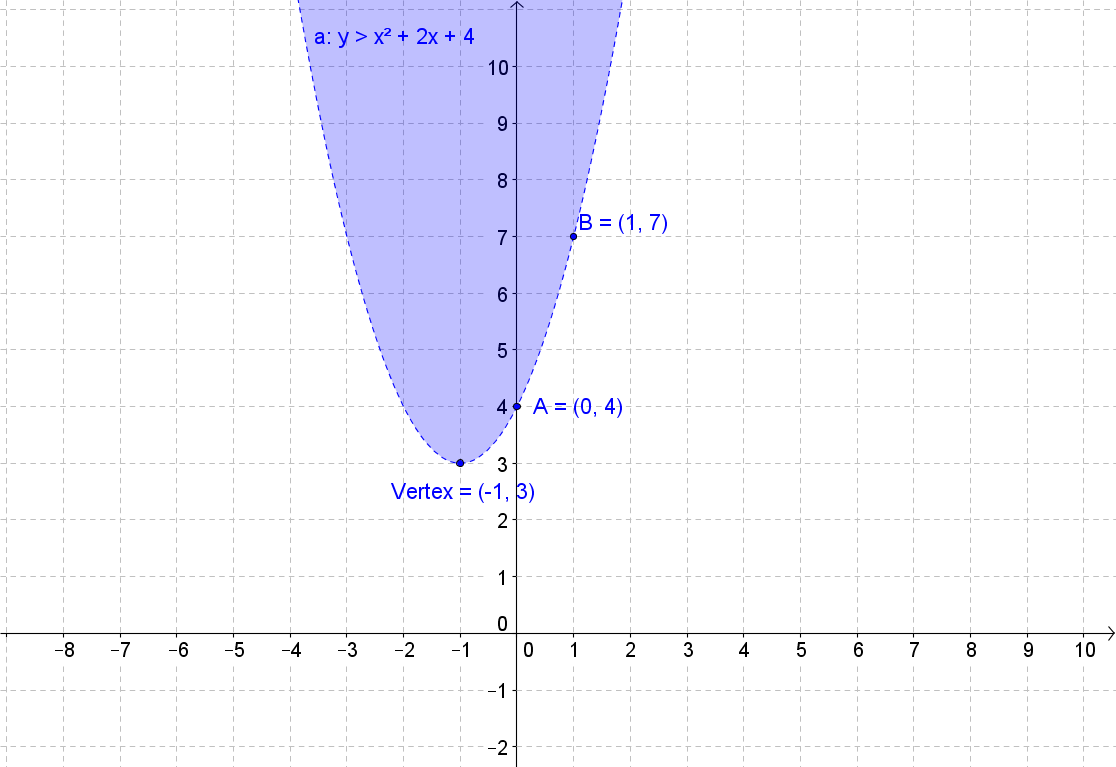Solve x2 − x − 6 > 0
• x2 − x − 6 = (x − 3)(x + 2) = 0
• Solve using Zero Product Property
• x − 3 = 0 and x + 2 = 0
• x = 3 and x = − 2
• Plot the roots and sketch the graph of the parabola given that a is > 0.
• Test the Inequality using a point not on the graph (0, 0)
• x2 − x − 6 > 0
• (0)2 − (0) − 6 > 0
• − 6 > 0
• Not True, which means that
• x <− 2
• x > 3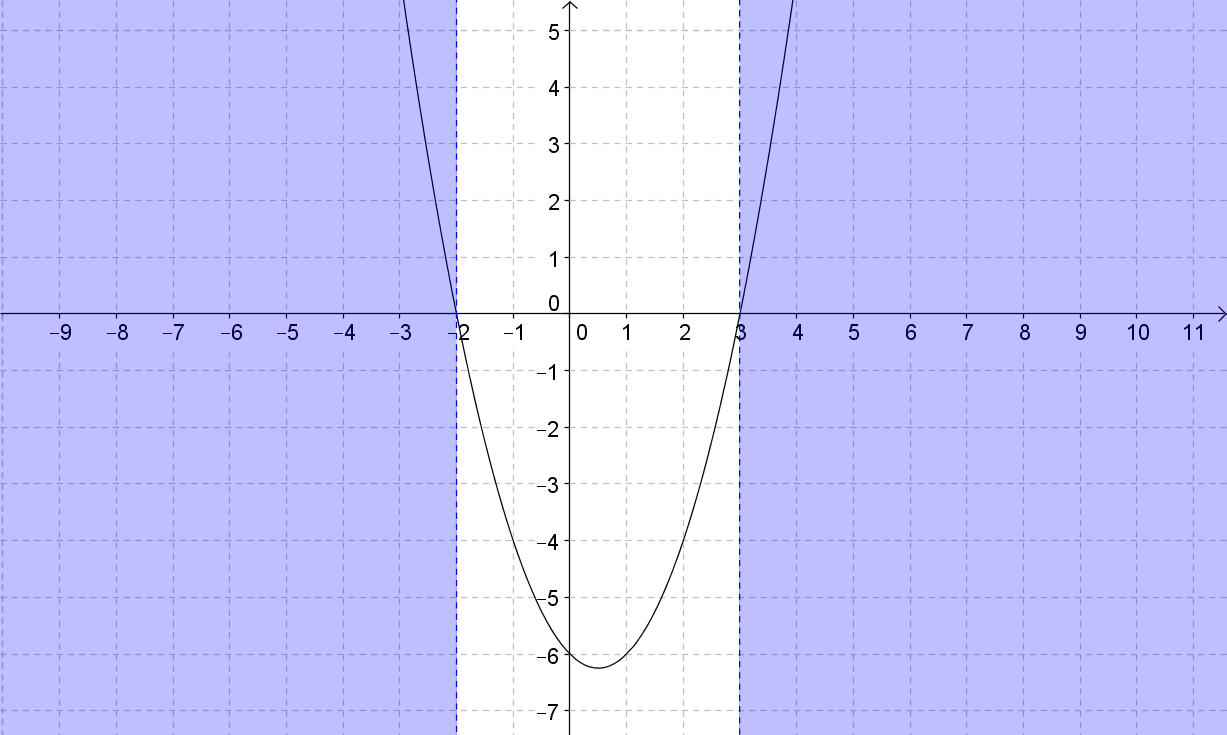Solve x2 + 5x + 4 < 0
• x2 + 5x + 4 = (x + 4)(x + 1) = 0
• Solve using Zero Product Property
• x + 4 = 0 and x + 1 = 0
• x = − 4 and x = − 1
• Plot the roots and sketch the graph of the parabola given that a is > 0.
• Test the Inequality using a point not on the graph (0, 0)
• x2 + 5x + 4 < 0
• (0)2 + 5(0) + 6 < 0
• 6 < 0
• Not True, which means that
• x >− 4 and
• x <− 1
• Can also be written as − 4 < x <− 3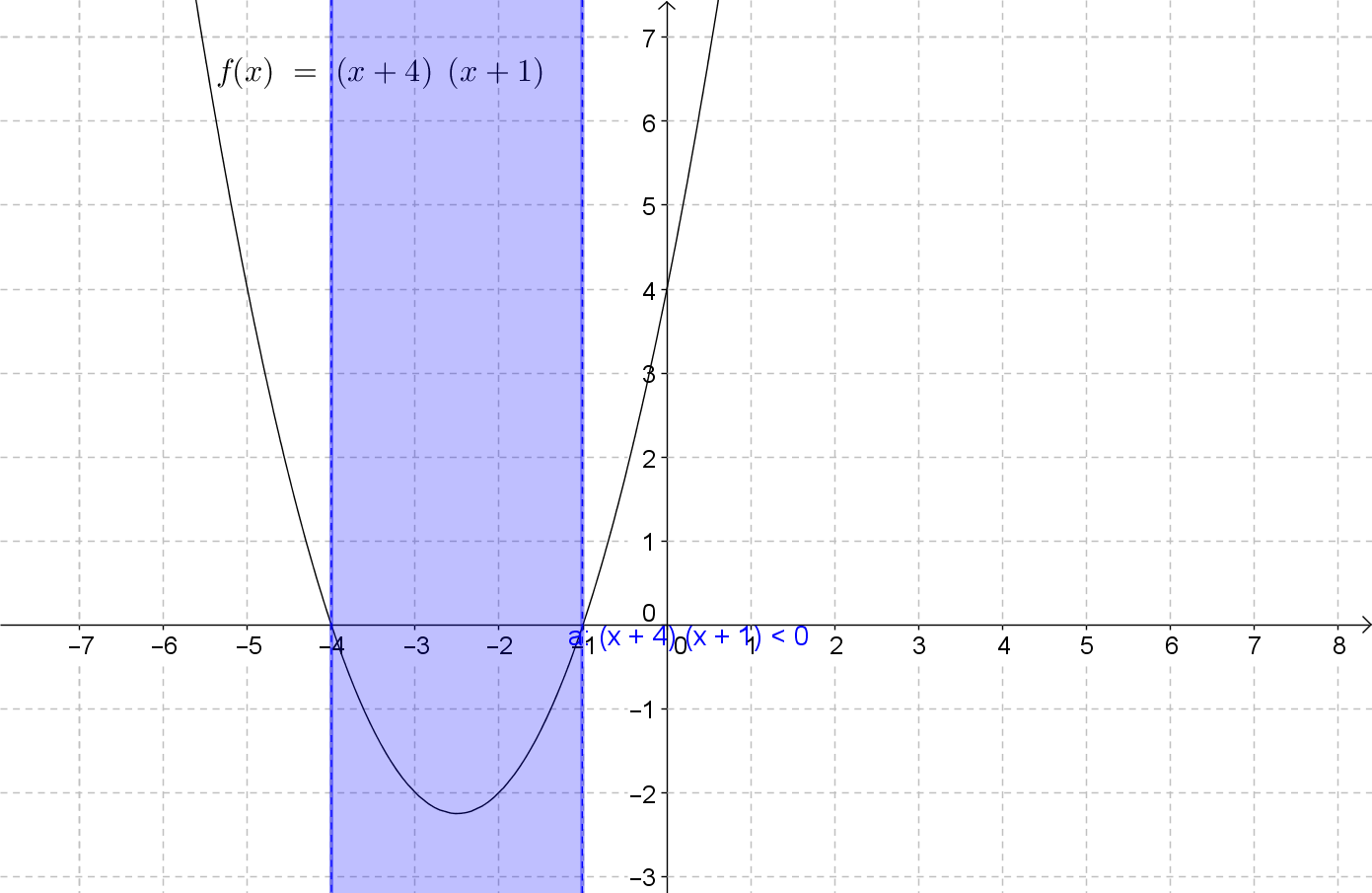Solve x2 − 8x + 7 > 0
• x2 − 8x + 7 = (x − 1)(x − 7) = 0
• Solve using Zero Product Property
• x − 1 = 0 and x − 7 = 0
• x = 1 and x = 7
• Plot the roots and sketch the graph of the parabola given that a is > 0.
• Test the Inequality using a point not on the graph (0, 0)
• x2 − 8x + 7 > 0
• (0)2 − 8(0) + 7 > 0
• 7 > 0
• True, which means that
• x < 1 or
• x > 7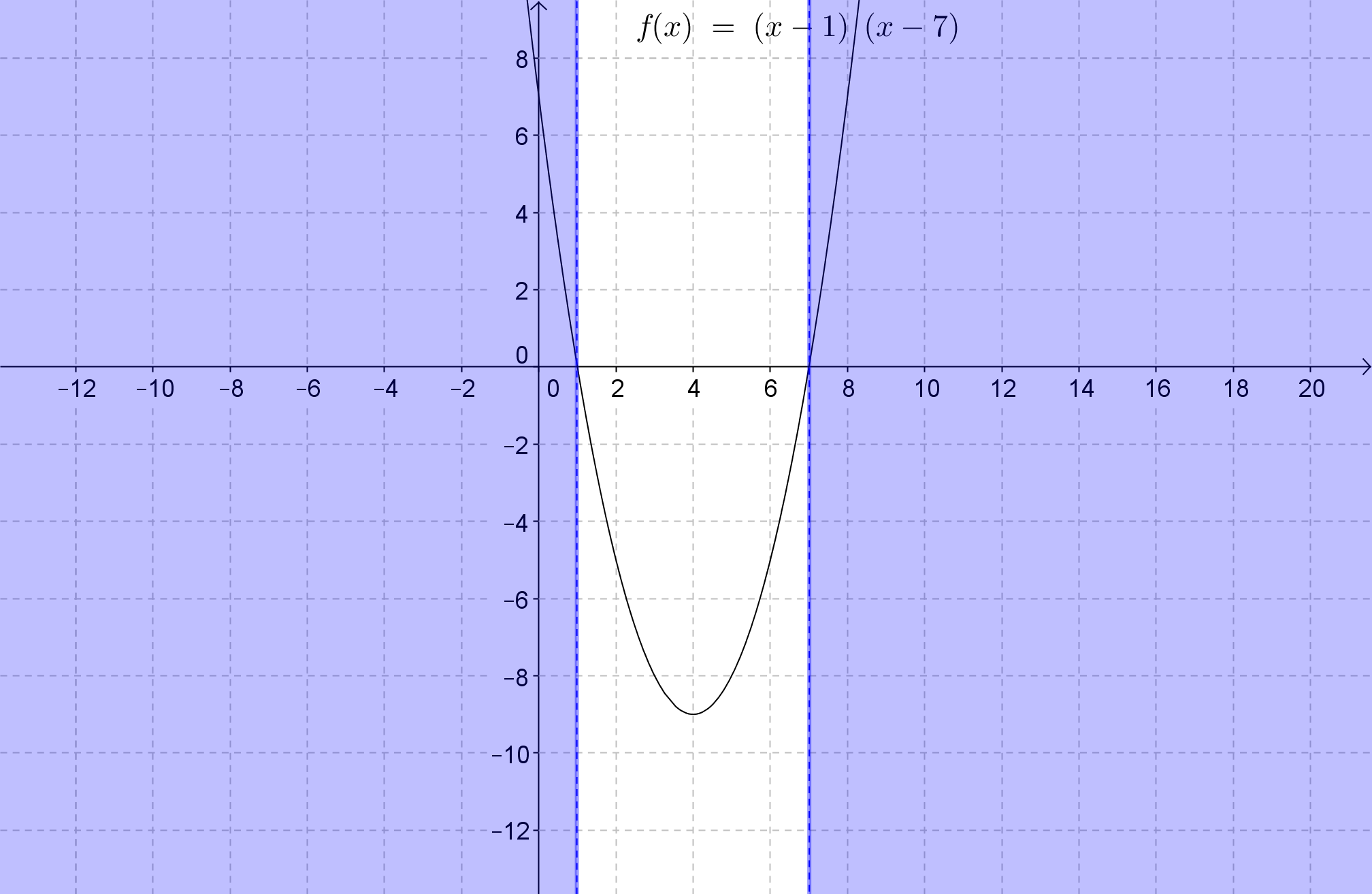Solve x2 − 4x + 3 > 0
• x2 − 4x + 3 = (x − 1)(x − 3) = 0
• Solve using Zero Product Property
• x − 1 = 0 and x − 3 = 0
• x = 1 and x = 3
• Plot the roots and sketch the graph of the parabola given that a is > 0.
• Test the Inequality using a point not on the graph (0, 0)
• x2 − 4x + 3 > 0
• (0)2 − 4(0) + 3 > 0
• 3 > 0
• True, which means that
• x < 1 or
• x > 3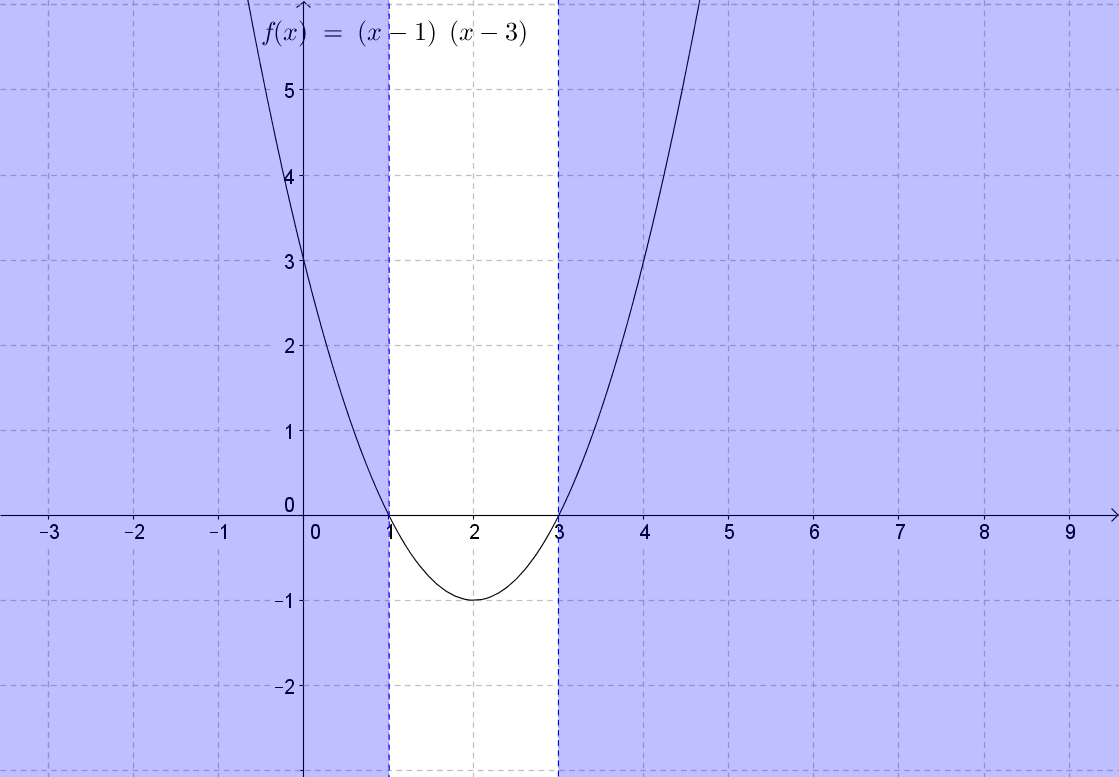Solve x2 − 2x − 15 < 0
• x2 − 2x − 15 = (x + 3)(x − 5) = 0
• Solve using Zero Product Property
• x + 3 = 0 and x − 5 = 0
• x = − 3 and x = 5
• Plot the roots and sketch the graph of the parabola given that a is < 0.
• Test the Inequality using a point not on the graph (0, 0)
• x2 − 2x − 15 < 0
• (0)2 − 2(0) − 15 < 0
• − 15 < 0
• True, which means that
• x >− 3 or
• x < 5
• Can also be written as − 3 < x < 5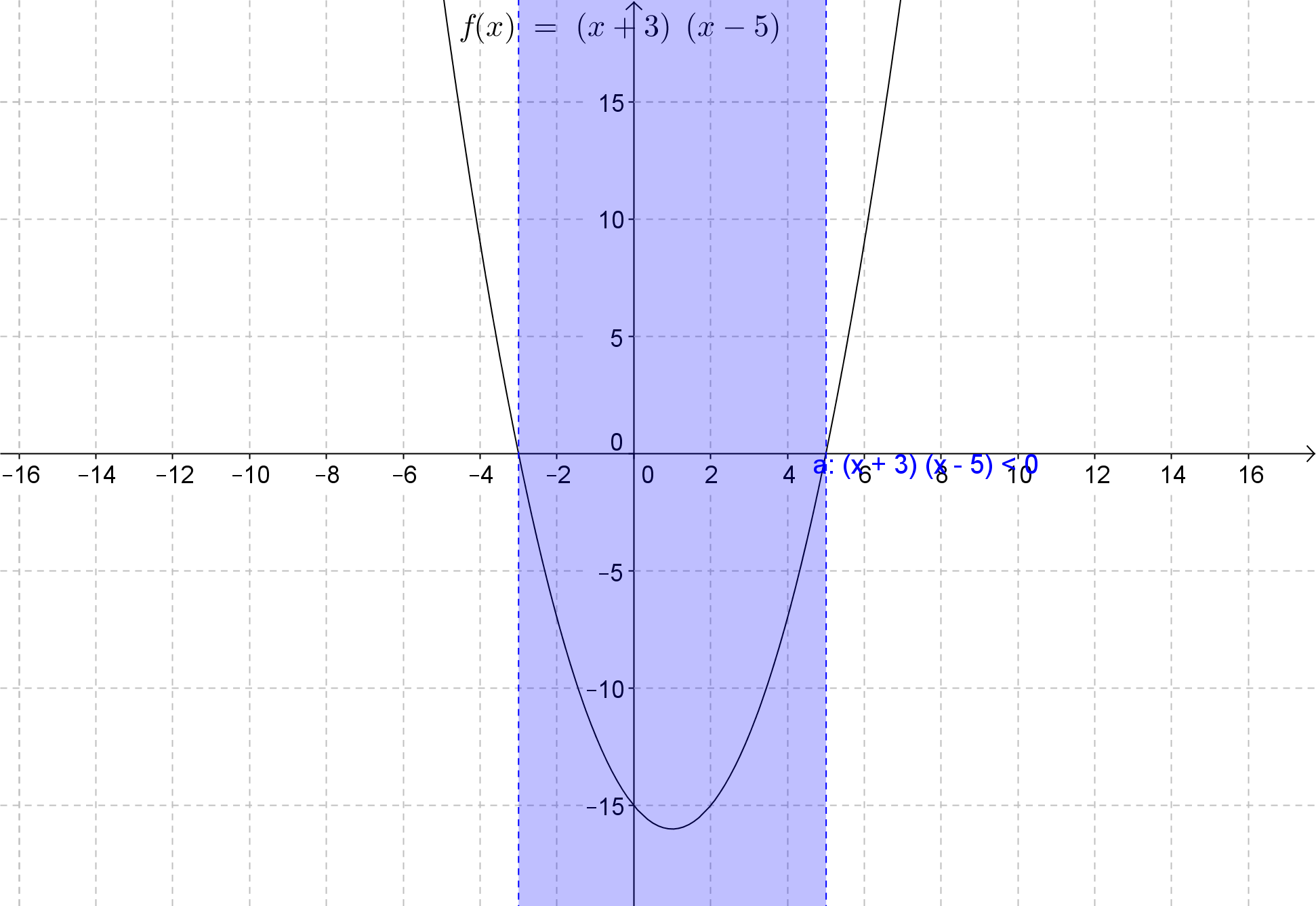*These practice questions are only helpful when you work on them offline on a piece of paper and then use the solution steps function to check your answer.

### Graphing and Solving Quadratic Inequalities

Lecture Slides are screen-captured images of important points in the lecture. Students can download and print out these lecture slide images to do practice problems as well as take notes while watching the lecture.

• Intro 0:00
• Test Point
• Example: Parameter
• Example 1: Graph Inequality 11:16
• Example 2: Solve Inequality 14:27
• Example 3: Graph Inequality 19:14
• Example 4: Solve Inequality 23:48

### Transcription: Graphing and Solving Quadratic Inequalities

Welcome to Educator.com.0000

Today, we are going to be talking about graphing and solving quadratic inequalities.0002

And we are going to apply some techniques learned from linear inequalities in our work.0006

So, in order to graph quadratic inequalities, use the techniques learned for graphing linear inequalities.0012

And recall: that involves graphing the related equation and using a test point,0018

except this time, we are going to be using a quadratic equation.0024

For example, just starting out with something simple: if you had an inequality y > x2 - 2,0029

the first step is to graph the related equation; and in this case, the related equation is going to be y = x2 - 2.0038

And that is going to give the boundary line for the solution set.0048

So, looking at this form, this is actually in vertex form; and here, a is 1; h is 0; and k is -2.0052

So, what this tells me is that the vertex of my graph is at (0,-2).0064

So, I have the vertex at (0,-2), right here.0071

And I also know that a is positive; a is greater than 0; so I know that the parabola opens upward.0074

So, I have a general idea of the shape of this graph before I even get started.0085

Now, to make a more exact graph, I am going to find some points.0089

And the first point is going to be -2, squared is 4, minus 2 is 2.0094

-1 squared is 1, minus 2 is -1; and then, I already have (0,-2); this is my vertex.0100

1 squared is 1, minus 2 is -1; and now, 2 squared is 4, minus 2 is 2.0110

This is enough for me to go ahead and graph.0119

(-2,2), (-1,-1), the vertex I already have graphed...actually, this goes -1, -1 is right there; and then (1,-1); (2,2).0122

So, I can see the shape of the graph.0146

Now, since this is a strict inequality, the boundary line is going to be dashed; it is not going to be part of the solution set.0148

And as expected, this parabola faces upward.0159

So, just as with linear inequalities, my first step is to graph the related equation.0163

My second step is to use a test point; and recall that a good test point to use is...I am going to use the test point (0,0),0167

because it is not near the boundary line, and it is a very easy point to use.0178

So, I am going to substitute (0,0) into this inequality, y > x2 - 2; so 0 is greater than 0 squared minus 2.0183

So, 0 is greater than -2; and that is true.0193

Since this is true, this test point is part of the solution set.0199

Now, the boundary line divides this into two sections: the area inside the parabola here, and the area outside the parabola.0205

And what the test point tells me is that, since the test point is inside the parabola, the solution set for the inequality is also inside the parabola.0214

If this had turned out to be not true, and the test point wasn't part of the solution set, then the solution set would have been out here.0223

OK, so that was graphing quadratic inequalities.0233

In this case, we are going to again graph the related quadratic function.0241

But then, we are going to find the roots of the related quadratic equation.0245

So, we are looking for something slightly different: remember, what I just looked at was this,0249

where I just had that y is greater than some function.0256

Now, this time, I am actually going to say something like x2 + x - 6 ≥ 0.0262

So, I actually have a number here; I have a parameter; I am saying that this function value is greater than or equal to a specific value.0270

So, I am going to start out by finding the roots, because by finding the roots, my graphing will be easier,0279

because recall that the roots give me the x-intercept.0289

Let's write the related quadratic--let's go ahead and find the roots by writing this as a quadratic equation.0300

And looking at this, as you know, there are many different ways to solve quadratic equations.0312

In this case, factoring would be an easy way; so I can see that I have a negative here, so I have x + something and x - something.0317

And I also know that my middle term is x; so I have to look at factors of 6, which are 1 and 6, and 2 and 3,0326

and look for a combination here that will add up to 1x.0338

And it actually turns out to be (x + 3) (x - 2), because that would give me -2x + 3x, to give me x for the middle term.0343

So, I did my factoring; and then, using the zero product property, I know that x + 3 = 0 and x - 2 = 0.0354

So, this gives me x = -3 and x = 2, so those are my roots: x = -3, and it can also equal 2.0363

That means that my x-intercepts are at -3 (this is -3) and 2; those are the roots.0380

OK, the next thing I am going to do in order to have my graph is find the vertex.0400

And recall that the vertex equals -b/2a; the x-value for the vertex equals -b/2a.0406

Here, I have that b is 1, so this is -1; a is also 1; so this is going to give me -1/2.0420

At the vertex, x equals -1/2; in order to find the y-value, I am going to substitute in.0430

And I am going to find out that I am going to get (-1/2)2 + 1/2 - 6.0441

So, I need to solve this to get my y-value; and this is going to give me 1/2...0455

this is actually -1/2 right here...(1/2)2 is going to give me 1/4, minus 1/2,0468

minus 6, which equals -1/4 - 6, which equals -6 and 1/4.0476

OK, so now I have my vertex; my vertex is at (-1/2, -6 1/4).0484

So, I can't graph that exactly; but 1, 2, 3, 4, 5, 6...-6 will be right here; and -1/2, -6 1/4 places it about there.0492

Now, I also know that, since a is positive (a is greater than 0), this is going to open upward.0509

So, I have my vertex here; and I know that this is going to open upward.0518

And this is greater than or equal to, so I can use a solid line; OK.0529

Now, what we are looking for, if we go back and think about this, is times when f(x), or y, is greater than or equal to 0.0536

I can use a test point, or I can just use logic.0546

I can look and see that here, at these x-intercepts, this is where y equals 0.0550

Beyond that, up here...it was when y equals 0; y equals 0 right here at x = -3, and y equals 0 at x = -2.0558

Beyond this point, for x-values over here, and for x-values over here, y is positive: y is greater than 0.0568

So, I can actually just look at this graph and say, "OK, when x is less than or equal to -3, or when x is greater than or equal to 2, this inequality is satisfied."0577

Now, you can always look at it and do it by logic; or you can use a test point.0597

So, if I use a test point of (0,0), what I would do is go back here and say,0603

"OK, I am going to let x equal 0; and I am going to see if that satisfies this inequality."0611

So, I am using my test point, and then I put it in; now I have 02 + 0 - 6 ≥ 0.0618

This gives me 0 - 6 ≥ 0; -6 ≥ 0.0628

That is not true--no; therefore, this is not part of the solution set.0633

These values in here are not part of the solution set; so that tells me that these are the correct values.0639

And I knew that by logic; but you can always use a test point or verify using a test point.0645

Therefore, my solutions for this inequality, for times when the function (or y) ends up0651

being greater than or equal to 0, are at points when x is less than or equal to -3, or greater than or equal to -2.0657

And you can see on the graph that that is true; when x is big over here, y is positive; when x is less than or equal to -3, y is also positive.0666

OK, first we are just asked to graph this inequality.0677

And we are going to begin by graphing the corresponding quadratic function in order to find the boundary line for the solution.0681

The corresponding function is y = x2 + 4.0692

This gives me an equation with a vertex at (0,4).0701

Now, let's find some points and then do the graphing.0710

When x is -2, this gives me 4 plus 4, or 8; when x is -1, this is 1, plus 4 is 5.0715

I already know that, when x is 0, y is 4, because that is the vertex.0726

And I know that this is a minimum, because the parabola opens upward, because a is positive.0731

So, a is greater than 0, so this opens upward.0736

And when x is 1, this is 1 plus 4; that gives me 5.0746

And when x is 2, that is 4 plus 4; that gives me 8.0751

So, 2, 4, 6, 8, 2, 4, 6, 8; let's start graphing, starting with the vertex at (0,4).0755

And I know this opens upward; and then, when x is -1, y is 5; when x is -2, y is 8.0768

When x is 1, y is 5; and when x is 2, y is 8.0781

So, because this is less than or equal to, I am going to use a solid line for this parabola.0788

And it is opening upward, as I expected.0794

Now, I graphed my corresponding quadratic function; I found this parabola; I graphed it;0798

and now, I need to use a test point--I am going to use the test point of (0,0) once again, because that is an easy point to use.0807

And I am going to go back here, and I am going to say y < x2 + 4.0818

0 is less than or equal to 0 squared plus 4; 0 is less than or equal to 0 plus 4; 0 ≤ 4.0824

And this is true: since this is true, the test point is part of the solution set;0832

and this parabola divides this graph into two sections--the area within the parabola and the area outside the parabola.0839

And since this is true, then my solution set lies out here.0847

So again, this is very similar to graphing linear inequalities, where you find the boundary line0852

by graphing the corresponding equation, and then use a test point to determine where the solution set is.0858

I am going to start out by finding the roots of the corresponding quadratic equation, x2 - x - 6 = 0.0872

This is also one that is most easily solved through factoring.0880

And since this term is negative, I am going to have x + something, and then x - something.0884

And I know that my factors of 6 are 1 and 6, and 2 and 3, and that I want these to sum up to -1.0892

1 and 6 are too far apart, so I look at 2 and 3.0901

And if I added -3 + 2, I would get -1; therefore, this is (x + 2) (x - 3).0905

And I can check that by saying, "OK, that would give me -3x + 2x, so my middle term would come out to -x."0913

OK, and this equals 0; using the zero product property, I get x + 2 = 0, and x - 3 = 0.0922

So, I solve and get x = -2 and x = 3.0934

So, my roots are right here: -2 and 3.0941

Something else I know is that this is going to open upward, because a is positive.0948

And I am going to go ahead and find the vertex, and that is -b/2a (that is the x-value for the vertex).0953

And that is going to give me negative...and b is -1/2, and a is 1.0964

So, that equals 1/2; so the vertex is at 1/2.0971

Now, to find the y-value, the x-value for the vertex (x = 1/2)--substitute that in to find y (the y point for the vertex).0977

That gives me (1/2)2 - 1/2 - 6.0994

This equals 1/4 - 1/2 - 6, which equals -1/2 - 6...actually, -1/4...that would be minus 2/4, and that would give me -1/4, minus 6 equals -6 1/4.1000

Therefore, the vertex lies at (1/2,-6 1/4).1022

Now I have the vertex, which is going to be right about down here.1033

And I know that this opens upward, and I know that it is going to be a solid line, because this is less than or equal to.1041

Now, what I want to know is, "What are the situations when y, or this function, is less than or equal to 0?"1069

And I can actually just look on here and say, "OK, y is less than or equal to 0 at all these values for x in here."1081

So, when x is less than or equal to 3, I see that the y becomes negative--dips below 0.1095

I could always use a test point of (0,0): substitute in (0,0) here; that would give me...1105

if my test point is (0,0), I am going to get 02 - 0 - 6 ≤ 0, or -6 ≤ 0.1113

And that is true; since that is true, this is part of the solution set.1122

And the solution set is comprised of x ≥ -2 and x < 3.1126

So, that is going to give me x ≥ -2 and ≤ 3.1137

So, that is my solution for this quadratic inequality.1150

OK, now graph the inequality: first graph the corresponding quadratic equation.1155

And I have y = x2 - x - 12, and I am going to start out by finding the vertex at -b/2a, which equals...1163

b is -1, so it is negative -1, over 2...a is 1; that is going to give me 1/2.1181

The x-value for the vertex is going to be 1/2; what is y going to be?1195

y is going to be (1/2)2 - 1/2 - 12, so y = -12 1/4.1202

So, 2, 4, 6, 8, 10, 12; the vertex is going to be here at 1/2, which is going to be about here, all the way down here.1222

And this parabola does open upward, because a is positive.1238

Now, I am going to go ahead and find some more points (in addition to the vertex) to graph.1243

So, when x is -2, if you figure this out, y ends up being -6.1248

When x is -1, this is going to give me 1 + 1; that is 2, so this will come out to -10.1255

When x is 0, that is going to give me -12.1263

When x is 1, that is going to be 1 - 1 is 0, minus 12; so that is going to be -12.1268

When x is 2, that is going to be 4 - 2 is 2, minus 12 is -10.1275

OK, graphing these: this is -2 right here; when x is -2, y is -6.1281

When x is -1, which is right about here, y is all the way down here at -10.1290

When x is 1, y is down here at -12, right about here; when x is 2, we get -10 right here.1297

So, I have a graph right here, and it is actually going to be a dashed line; it is going to give me a parabola that is a dashed line,1315

because I have greater than; so, my boundary line is not part of the solution set.1335

So now, I have this parabola; and I need to use a test point right here.1342

Test point (0,0): substituting in here is going to give me 0 > 02 - 0 - 12.1346

0 is greater than 0 - 12; 0 is greater than -12.1357

OK, so is 0 greater than -12? Yes, therefore, since 0 is greater than -12, this point would be part of the solution set.1366

So, the solution set is going to be in here.1386

Again, we handled this much the way we would handle a linear inequality.1392

And that is by graphing out the corresponding equation, y = x2 - x - 12.1398

And it gave me this parabola, with a vertex way down here that is a minimum that opens upward.1405

Then, I went and found a test point, (0,0), and I substituted this in and came up with 0 > -12.1410

And 0 actually is greater than -12, so this test point is part of the solution set, which would be right here within the parabola.1420

Now, solve this quadratic inequality; first I am going to go ahead and find the roots,1429

which are of the corresponding quadratic equation.1435

And this is another one that I can do by factoring; I am going to have x + something, and I am going to have x - something.1443

And I know that, because I have a negative 10; so I am going to have a positive and a negative.1451

Now, the factors of 10 are 1 and 10, and 2 and 5; and I need something that is going to add up to 3x.1456

And what would give me that is if I had a positive 5 and a negative 2, because then I am going to get -2x + 5x, to get 3x.1464

Using the zero product property, I will have x + 5 = 0, or x = -5; x - 2 = 0 gives me x = 2.1475

So, I have two roots that I found; and these are x = -5 and x = 2: right here and right here.1486

Now, I could find the vertex, and I have been finding it; but you actually don't need it for these.1498

All you need to know is that the zeroes are here, and this graph opens upward.1505

So, I am just going to sketch this out--that this is a parabola that opens upward,1510

and I don't know exactly where the vertex is; that is OK, because I am honestly not concerned with that.1526

What I am concerned with are these points right here.1532

And I am looking for values of x where this function would end up being greater than 0.1534

So, I am going to look over here and see that, whenever x is less than -5, y is greater than 0.1542

Whenever x is greater than 2, y is also positive.1550

I could also use my test point; and again, I use the origin as a test point, as long as it is not too close to the boundary.1559

So, my test point is (0,0); and now I am going to use the x-value and put it in here and find that 02 + 3 times 0 - 10 > 0.1568

Let's see if that is true: 0 + 0 - 10 > 0--is -10 greater than 0? No.1579

So, this test point is not part of the solution set; the solution set is not in here--it is out here, just as I thought.1587

So, what this gives me is a solution set where x is less than -5, or x is greater than 2.1593

So, my solution set are values of x that are less than -5 or greater than 2.1609

That concludes this session of Educator.com on quadratic inequalities.1619

And I will see you next lecture!1624

OR

### Start Learning Now

Our free lessons will get you started (Adobe Flash® required).